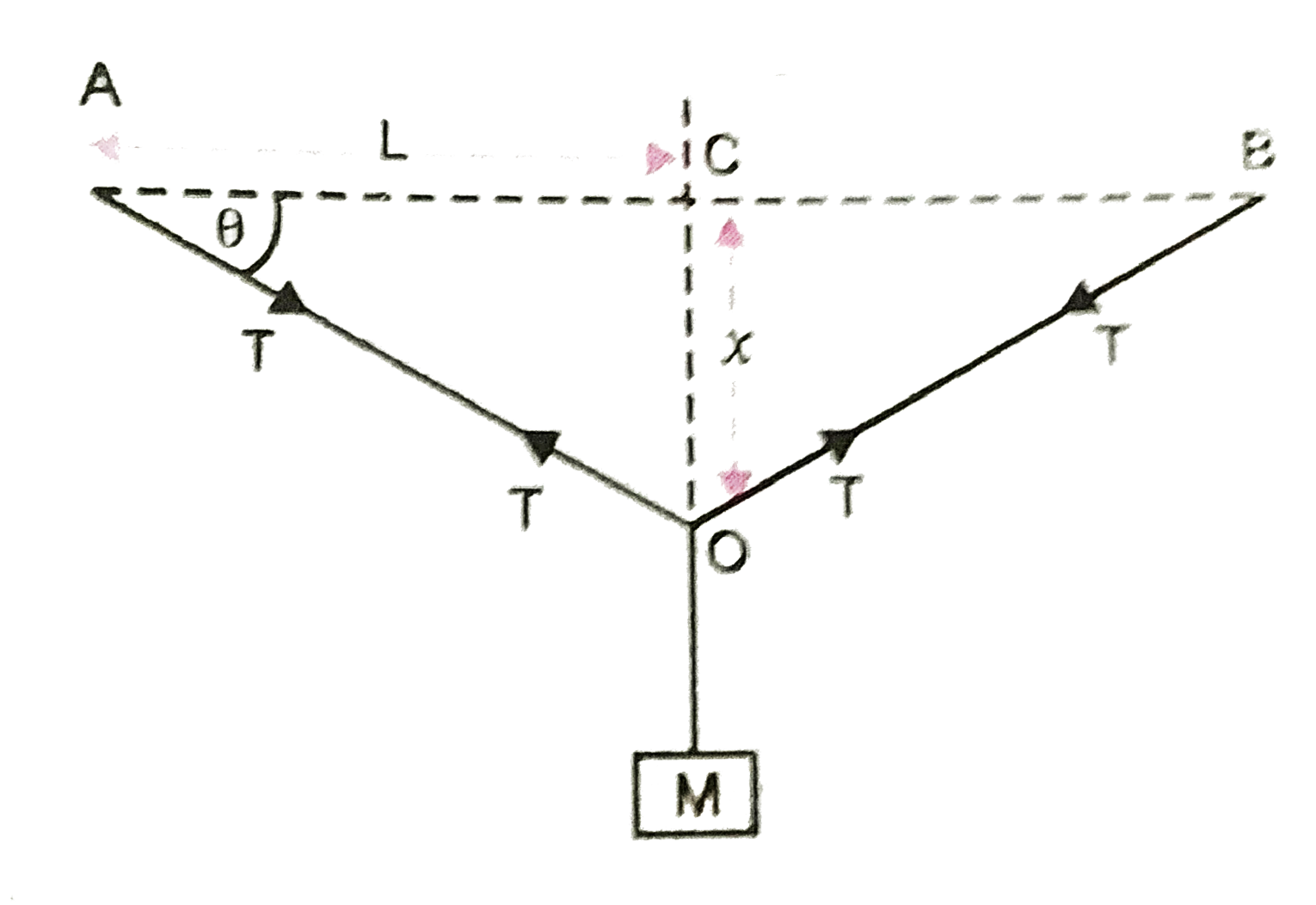# A wire of cross sectional area 4xx10^(-4) m^2 modulus of rigidity 2xx10^(11) N//m^2 and length 1 m is stretched between two vertical rigid poles.

17 views
in Physics
closed
A wire of cross sectional area 4xx10^(-4) m^2 modulus of rigidity 2xx10^(11) N//m^2 and length 1 m is stretched between two vertical rigid poles. A mass of 1kg is suspended at its middle. Calculate the angle it makes with horizontal. Given g = 10 ms^(-2)

by (90.5k points)
selected

Refer tofor equilibrium position of mass M, Mg = 2T sin theta…… (i)
if theta is small, sin theta ~~ theta = x//L….. (ii)
As, T = (YA)/(L) Delta L = (YA)/(L)[(L^2 + x^2)^(1//2)-L]
From (i) Mg = (2YA x^2)/(2L^2) xx sin theta = YA theta^2 xx theta = YA theta^3
or theta = ((Mg)/(YA))^(1//3) = [(1xx10)/(2xx 10^(11)xx4 xx10^(-4))]^(1//3)
~~ 0.005 rad